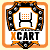View Single Post
#3cherieX-Wizard Join Date: May 2003 Location: USA Posts: 1,532Here's what we just implemented. This is with 4.0.14. Our conditions are payment method "Checks/Money Orders" and non-Wholesale customers.

1. Pass the paymentid by changing cart.php around line 587 from:
Code:
```#
# Calculate all prices
#
\$cart = func_array_merge (\$cart, func_calculate(\$cart, \$products, \$login, \$current_area));```
to:
Code:
```#
# Calculate all prices
#
\$cart = func_array_merge (\$cart, func_calculate(\$cart, \$products, \$login, \$current_area, \$paymentid));```

2. Accept the paymentid by changing include/func.php around line 1007 from:
Code:
`function func_calculate(\$cart, \$products, \$login, \$login_type) {`
to:
Code:
`function func_calculate(\$cart, \$products, \$login, \$login_type, \$paymentid="") {`

3. In the same file and function, pass the paymentid again by changing the following around line 1019:
Code:
`\$result = func_calculate_single (\$cart, \$products, \$login, \$login_type);`
to:
Code:
`\$result = func_calculate_single (\$cart, \$products, \$login, \$login_type, "", \$paymentid);`
and around line 1034 from:
Code:
`\$result = func_calculate_single (\$cart, \$_products, \$login, \$login_type, \$provider_for);`
to:
Code:
`\$result = func_calculate_single (\$cart, \$_products, \$login, \$login_type, \$provider_for, \$paymentid);`
and around line 1074 from:
Code:
`\$result = func_calculate_single (\$cart, \$_products, \$login, \$login_type);`
to:
Code:
`\$result = func_calculate_single (\$cart, \$_products, \$login, \$login_type, "", \$paymentid);`
These last two changes may not be necessary.

4. In the same file, adjust function func_calculate_single to accept the paymentid by changing:
Code:
`function func_calculate_single(\$cart, \$products, \$login, \$login_type, \$provider_for="") {`
to:
Code:
`function func_calculate_single(\$cart, \$products, \$login, \$login_type, \$provider_for="", \$paymentid) {`

5. In the same file and function, add the following towards the end of the function:
Code:
```if ( (\$customer_info[membership] != "Wholesale") && (\$paymentid == 5) ) {
\$discounted_subtotal -= (\$subtotal * .05);
\$display_discounted_subtotal = \$discounted_subtotal;
}```
just above:
Code:
```#
#```
Your paymentid may be different as well as the exact membership name.

6. Adjust skin1/customer/main/cart_totals.tpl by adding the following after the if block for "if \$cart.discount gt 0":
Code:
```{if ( (\$userinfo.membership ne "Wholesale") and (\$smarty.get.paymentid eq 5) )}
{math equation="x*.05" assign="payment_discount" x=\$cart.subtotal}
<tr>
<td nowrap><font class="FormButton">Payment Discount:</font></td>
<td>[img]{\$ImagesDir}/null.gif[/img]</td>
<td nowrap align="right"><font class="ProductPriceSmall">{include file="currency.tpl" value=\$payment_discount}</font></td>
<td nowrap align="right">{include file="customer/main/alter_currency_value.tpl" alter_currency_value=\$payment_discount}</td>
</tr>
{/if}```

To also display this information in the order history and invoice:

7. Add the following to function func_order_data in include/func.php towards the bottom just above the return:
Code:
```if ( (\$order[membership] != "Wholesale") && (\$order[payment_method] == "Checks/Money Orders") ) {
\$order[discounted_subtotal] -= (\$order[subtotal] * .05);
\$order[display_discounted_subtotal] = \$order[discounted_subtotal];
}```

8. Add the following to skin1/mail/html/order_data.tpl after the if block for "if \$order.discount gt 0":
Code:
```{if ( (\$order.membership ne "Wholesale") and (\$order.payment_method eq "Checks/Money Orders") )}
{math equation="x*.05" assign="payment_discount" x=\$order.subtotal}
<tr>
<td align="right" height="20">Payment Discount:</TD>
<td align="right">{include file="currency.tpl" value=\$payment_discount}</td>
</tr>
{/if}```
Your exact payment method name may be different.

If anyone has any improvements to this, please post.__________________
redlimeweb.com